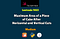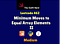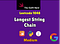# Swift Leetcode Series: Maximum Area of a Piece of Cake After Horizontal and Vertical Cuts

## Swift Solution for Leetcode 1465The Swift Nerd

You can read the full story on The Swift Nerd blog with the link above.

# Problem Statement

Given a rectangular cake with height `h` and width `w`, and two arrays of integers `horizontalCuts` and `verticalCuts` where `horizontalCuts[i]` is the distance from the top of the rectangular cake to the `ith` horizontal cut and similarly, `verticalCuts[j]` is the distance from the left of the rectangular cake to the `jth` vertical cut.

Return the maximum area of a piece of cake after you cut at each horizontal and vertical position provided in the arrays `horizontalCuts` and `verticalCuts`.

# Swift Leetcode Series: Max Area of Island

## Swift + DFS + Recursion ✅ ✅ ✅

You can read the full story on The Swift Nerd blog with the link above.

# Problem Description

You are given an `m x n` binary matrix `grid`. An island is a group of `1`'s (representing land) connected 4-directionally (horizontal or vertical.) You may assume all four edges of the grid are surrounded by water.

The area of an island is the number of cells with a value `1` in the island.

Return the maximum area of an island in `grid`. If there is no island, return `0`.

Example 1:

# Swift Leetcode Series: Evaluate Reverse Polish Notation

## Stacks + Expressions = Leetcode 150 🚀 🚀 🚀

Read the full story on The Swift Nerd blog with the link above.

# Problem Description

Evaluate the value of an arithmetic expression in Reverse Polish Notation.

Valid operators are `+`, `-`, `*`, and `/`. Each operand may be an integer or another expression.

Note that division between two integers should truncate toward zero.

It is guaranteed that the given RPN expression is always valid. That means the expression would always evaluate to a result, and there will not be any division by zero operation.

# Examples

`Input: tokens = ["2","1","+","3","*"]Output: 9Explanation: ((2 + 1) * 3) = 9Input: tokens = ["4","13","5","/","+"]Output…`

# Find and Replace Pattern

## Swift + Pattern Matching = 🧠🛠️🚀

Check out the full story on The Swift Nerd blog with the link above.

# Problem Description

Given a list of strings `words` and a string `pattern`, return a list of `words[i]` that match `pattern`. You may return the answer in any order.

A word matches the pattern if there exists a permutation of letters `p` so that after replacing every letter `x` in the pattern with `p(x)`, we get the desired word.

Recall that a permutation of letters is a bijection from letters to letters: every letter maps to another letter, and no two letters map to the same letter.

# Examples

`Input: words…`

# Swift Leetcode Series: Binary Tree Level Order Traversal

## Traverse Trees with Swift like a Pro 🚀 🚀 🚀

You can read the full story on The Swift Nerd blog with the link above.

# Problem Description

Given the `root` of a binary tree, return the level order traversal of its nodes’ values. (i.e., from left to right, level by level).

# Swift Leetcode Series: Minimum Moves to Equal Array Elements

## Solve Leetcode Challenges in Swift like a ProTheSwiftNerd

You can read the full story on The Swift Nerd blog with the link above.

# Problem Description

Given an integer array `nums` of size `n`, return the minimum number of moves required to make all array elements equal.

In one move, you can increment or decrement an element of the array by `1`.

# Examples

`Input: nums = [1,2,3]Output: 2Explanation:Only two moves are needed (remember each move increments or decrements one element):[1,2,3]  =>  [2,2,3]  =>  [2,2,2]Input: nums = [1,10,2,9]Output: 16`

# Constraints

• `n == nums.length`
• `1 <= nums.length <= 105`
• `-109 <= nums[i] <= 109`

# Solution

One brute force approach can…

# Google Interview Problem: Longest String Chain

## Swift + Dynamic Programming = 🔥 🔥 🔥TheSwiftNerd: Leetcode 1048

If you are preparing for coding interviews, then check the amazing Swift Leetcode series on The Swift Nerd blog.

# Problem Description

Given a list of words, each word consists of English lowercase letters.

Let’s say `word1` is a predecessor of `word2` if and only if we can add exactly one letter anywhere in `word1` to make it equal to `word2`. For example, `"abc"` is a predecessor of `"abac"`.

A word chain is a sequence of words `[word_1, word_2, ..., word_k]` with `k >= 1`, where `word_1` is a predecessor of `word_2`, `word_2` is a predecessor of `word_3`, and so on.

Return the…

# Swift Leetcode Series: Valid Number

## Facebook Interview Question 👍 👍 👍

You checkout the full story on The Swift Nerd blog with the link above.

# Problem Description

A valid number can be split up into these components (in order):

1. A decimal number or an integer.
2. (Optional) An `'e'` or `'E'`, followed by an integer.

A decimal number can be split up into these components (in order):

1. (Optional) A sign character (either `'+'` or `'-'`).
2. One of the following formats:
3. At least one digit, followed by a dot `'.'`.
4. At least one digit, followed by a dot `'.'`, followed by at least one digit.
5. A dot `'.'`, followed by at least one digit.

An…

# Swift Leetcode Series: Flatten Binary Tree to Linked List

## Swift + Binary Trees + Linked List = Leetcode 114 ✅✅✅

Check the full story on The Swift Nerd blog with the link above.

# Problem Description

Given the `root` of a binary tree, flatten the tree into a “linked list”:

• The “linked list” should use the same `TreeNode` class where the `right` child pointer points to the next node in the list and the `left` child pointer is always `null`.
• The “linked list” should be in the same order as a pre-order traversal of the binary tree.

# Swift Leetcode Series: Interval List Intersections

## Merge intervals in Swift like a Pro

You can read the full story on The Swift Nerd blog along with other interesting ones.

# Problem Statement

You are given two lists of closed intervals, `firstList` and `secondList`, where `firstList[i] = [starti, endi]` and `secondList[j] = [startj, endj]`. Each list of intervals is pairwise disjoint and in sorted order.

Return the intersection of these two interval lists.

A closed interval `[a, b]` (with `a < b`) denotes the set of real numbers `x` with `a <= x <= b`.

The intersection of two closed intervals is a set of real numbers that are either empty or represented as a closed interval…## Varun

Senior iOS Engineer. Writer at https://theswiftnerd.com